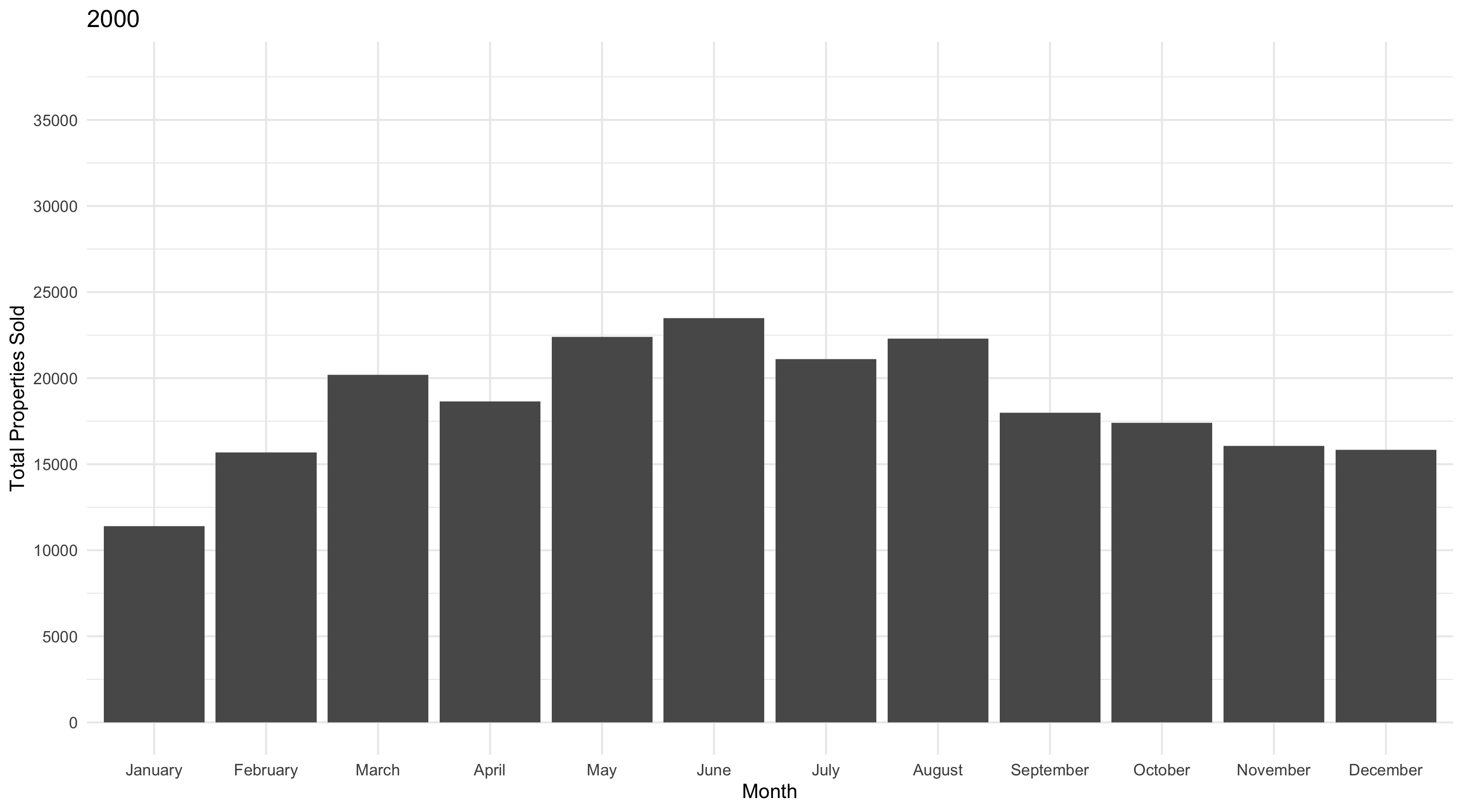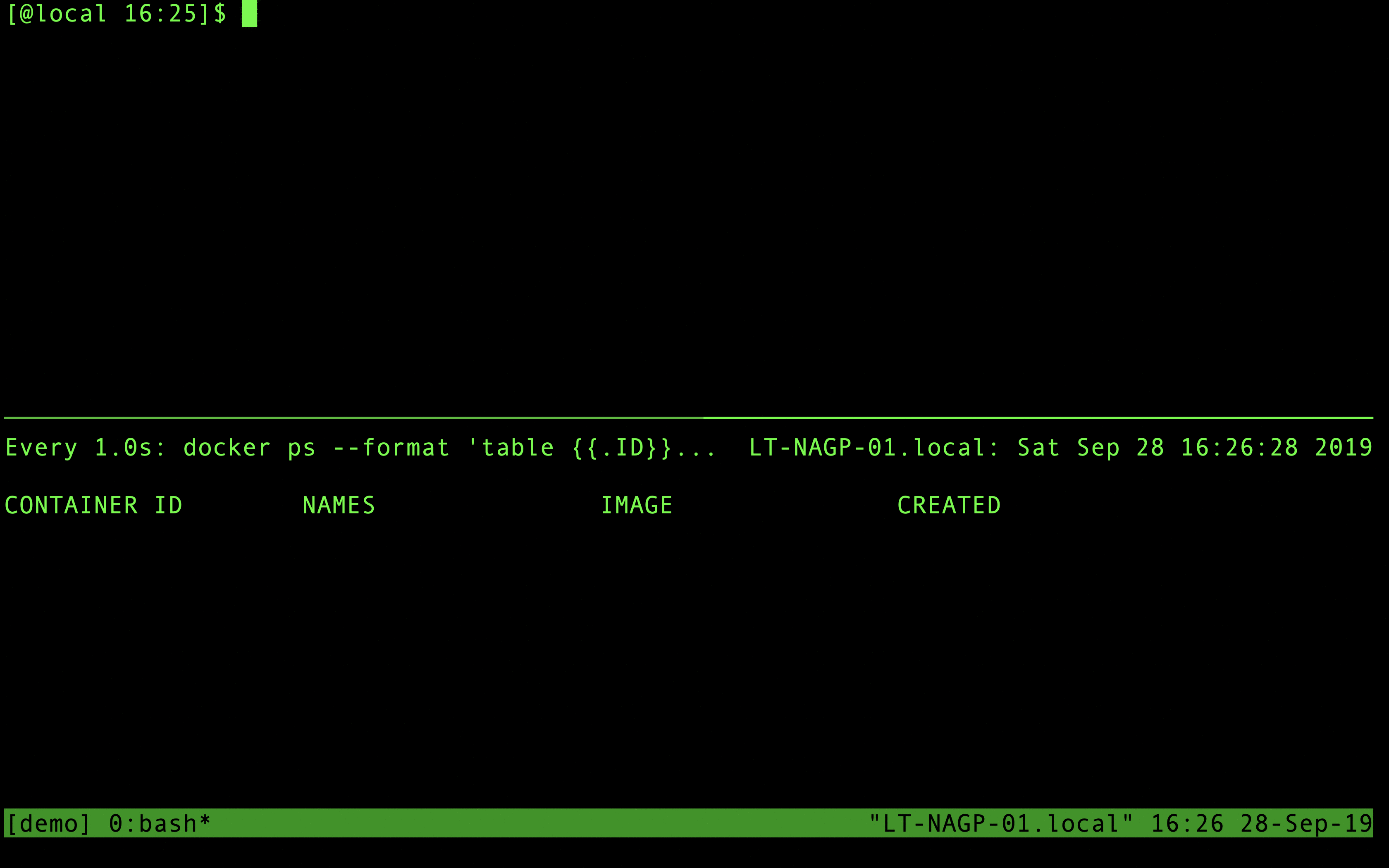# Create a GIF with R

With R you can turn a collection of images into an animated GIF. That can be useful for animating plots or for converting a series of arbitrary image files (not created in R) into an animation. The following will include examples of both use-cases, with a reproducible demo of the former.

The tool used in the example that follows is the `magick` R package, which is a wrapper for the ImageMagick library.

The first example involves animating plots that are created in R. To motivate this example we’re using a built-in dataset from ggplot2 (`txhousing`), which details historical residential property sales/listings in Texas by county between 2000-2015:

``head(ggplot2::txhousing)``
``````## # A tibble: 6 x 9
##   city     year month sales   volume median listings inventory  date
##   <chr>   <int> <int> <dbl>    <dbl>  <dbl>    <dbl>     <dbl> <dbl>
## 1 Abilene  2000     1    72  5380000  71400      701       6.3 2000
## 2 Abilene  2000     2    98  6505000  58700      746       6.6 2000.
## 3 Abilene  2000     3   130  9285000  58100      784       6.8 2000.
## 4 Abilene  2000     4    98  9730000  68600      785       6.9 2000.
## 5 Abilene  2000     5   141 10590000  67300      794       6.8 2000.
## 6 Abilene  2000     6   156 13910000  66900      780       6.6 2000.``````

The code below will prepare the data for plotting, then loop through all of the 16 years in the dataset and create barplots of total sales each month for every year. These plots will be written to disk as static `.png` files.

``````library(magick)
library(ggplot2)
library(dplyr)
library(tidyr)

## create a directory to which the images will be written
dir_out <- file.path(tempdir(), "tx-sales")
dir.create(dir_out, recursive = TRUE)

## prepare data
tx_sales <-
txhousing %>%
group_by(year,month) %>%
summarise(sales = sum(sales, na.rm = TRUE)) %>%
ungroup() %>%
mutate(month = factor(month, labels = month.name)) %>%
complete(month,year)

## get a sorted list of unique years in the TX housing dataset
years <-
tx_sales %>%
pull(year) %>%
unique(.) %>%
sort(.)

## find the month with the most houses sold to set y axis limit
most_sold <- max(tx_sales\$sales, na.rm = TRUE)

## loop through years ...
## subset data ...
## create barplot of sales by month for each year ...
## write plot to file
for (y in years) {

p <-
tx_sales %>%
filter(year == y) %>%
ggplot(aes(month,sales)) +
geom_col() +
scale_y_continuous(limits = c(0, most_sold), breaks = seq(0,1e5, by = 5000)) +
theme_minimal() +
labs(x = "Month", y = "Total Properties Sold", title = y)

fp <- file.path(dir_out, paste0(y, ".png"))

ggsave(plot = p,
filename = fp,
device = "png")

}``````

With the plots written to disk (there should be 16 `.png` files in this example), we can now use `magick` to read in the image data and stitch it all together in an animation.

``````## list file names and read in
imgs <- list.files(dir_out, full.names = TRUE)

## join the images together
img_joined <- image_join(img_list)

## animate at 2 frames per second
img_animated <- image_animate(img_joined, fps = 2)

## view animated image
img_animated

## save to disk
image_write(image = img_animated,
path = "tx-sales.gif")``````To be clear … the example above is contrived to reproducibly demonstrate the `magick` functionse.

Another (maybe more straightforward) approach to the example outlined above would be to use `gganimate`:

``````library(gganimate)

tx_sales %>%
ggplot(aes(month,sales)) +
geom_col() +
scale_y_continuous(limits = c(0, most_sold), breaks = seq(0,1e5, by = 5000)) +
theme_minimal() +
## gganimate functionality starts here
labs(x = "Month", y = "Total Properties Sold", title = "{frame_time}") +
transition_time(year) +
ease_aes("linear")

anim_save("tx-sales-gganimate.gif")``````But note that these GIFs are not identical. `gganimate` transitions the the plot between years in a way that makes the bars expand and contract smoothly. That may or may not be the desired effect. `ease_aes()` can customize this behavior but it will likely be different than the GIF created with `magick`.

More importantly, the `magick` method for creating a GIF can be extended to cases when the images are not created in R. For example, the GIF below was created using a similar set of steps (`image_read() %>% image_join() %>% image_animate()`) on list of screenshots.